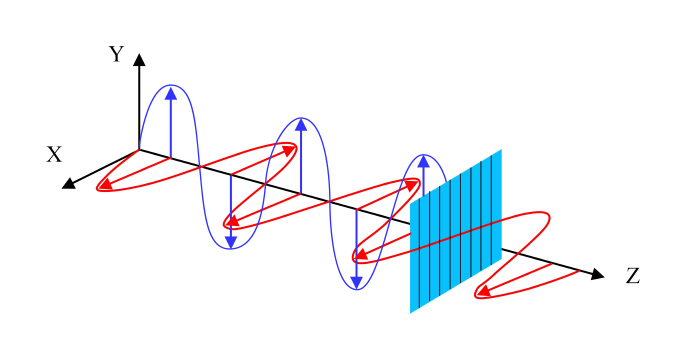偏振片是可以使自然光变成偏振光的光学元件，对入射光具有遮蔽和透过的功能。大部分晶体在自然光入射的情况下产生的o光和e光的强度相等。但是，也有一些晶体对两支折射光的吸收相差很大，这种性质叫做二向色性。利用晶体的二向色性可以制作偏振片。一个自然光的平行光束可看作是由在垂直于传播方向的任何两个相互正交方向上偏振的两个互不相干的等振幅光束组成。选择偏振片中主振动方向作为这两部分光束的振动方向，如果$$E$$ 是进入偏振片的两个部分光束中每一个的振幅，则在偏振片中传播距离$$L$$ 后、它们的振幅为：

$E_{1}=E\exp^{-k_{1}\omega_{1}}/v$ $E_{2}=E\exp^{-k_{2}\omega_{1}}/v$

$E_{1}/E_{2}=\exp ^{-\left ( k_{1}-k_{2}\right )\omega _{1}/v}$